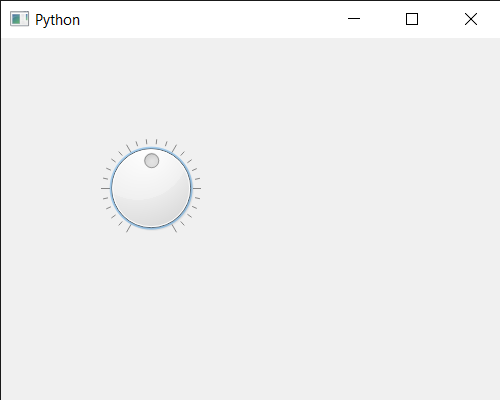# PyQt5 QDial – Setting Slider’s Value

• Last Updated : 07 Jul, 2020

In this article we will see how we can set slider’s value of the QDial. User can change the value with the help of mouse although we can change it programmatically as well. The slider forces the value to be within the legal range i.e value should be greater than or equal to the minimum value and it should be less than or equal to the maximum limit. Changing the value also changes the slider position.

In order to do this we use `setValue` method with the QDial object

Syntax : dial.setValue(n)

Argument : It takes integer as argument

Return : It returns None

Below is the implementation

 `# importing libraries``from` `PyQt5.QtWidgets ``import` `*` `from` `PyQt5 ``import` `QtCore, QtGui``from` `PyQt5.QtGui ``import` `*` `from` `PyQt5.QtCore ``import` `*` `import` `sys`` ` ` ` `class` `Window(QMainWindow):`` ` `    ``def` `__init__(``self``):``        ``super``().__init__()`` ` `        ``# setting title``        ``self``.setWindowTitle(``"Python "``)`` ` `        ``# setting geometry``        ``self``.setGeometry(``100``, ``100``, ``500``, ``400``)`` ` `        ``# calling method``        ``self``.UiComponents()`` ` `        ``# showing all the widgets``        ``self``.show()`` ` `    ``# method for components``    ``def` `UiComponents(``self``):`` ` `        ``# creating QDial object``        ``dial ``=` `QDial(``self``)`` ` `        ``# setting geometry to the dial``        ``dial.setGeometry(``100``, ``100``, ``100``, ``100``)`` ` `        ``# making notch visible``        ``dial.setNotchesVisible(``True``)`` ` `        ``# value``        ``value ``=` `50`` ` `        ``# setting value to the dial slider``        ``dial.setValue(value)`` ` ` ` ` ` ` ` `# create pyqt5 app``App ``=` `QApplication(sys.argv)`` ` `# create the instance of our Window``window ``=` `Window()`` ` `# start the app``sys.exit(App.``exec``())`

Output :My Personal Notes arrow_drop_up